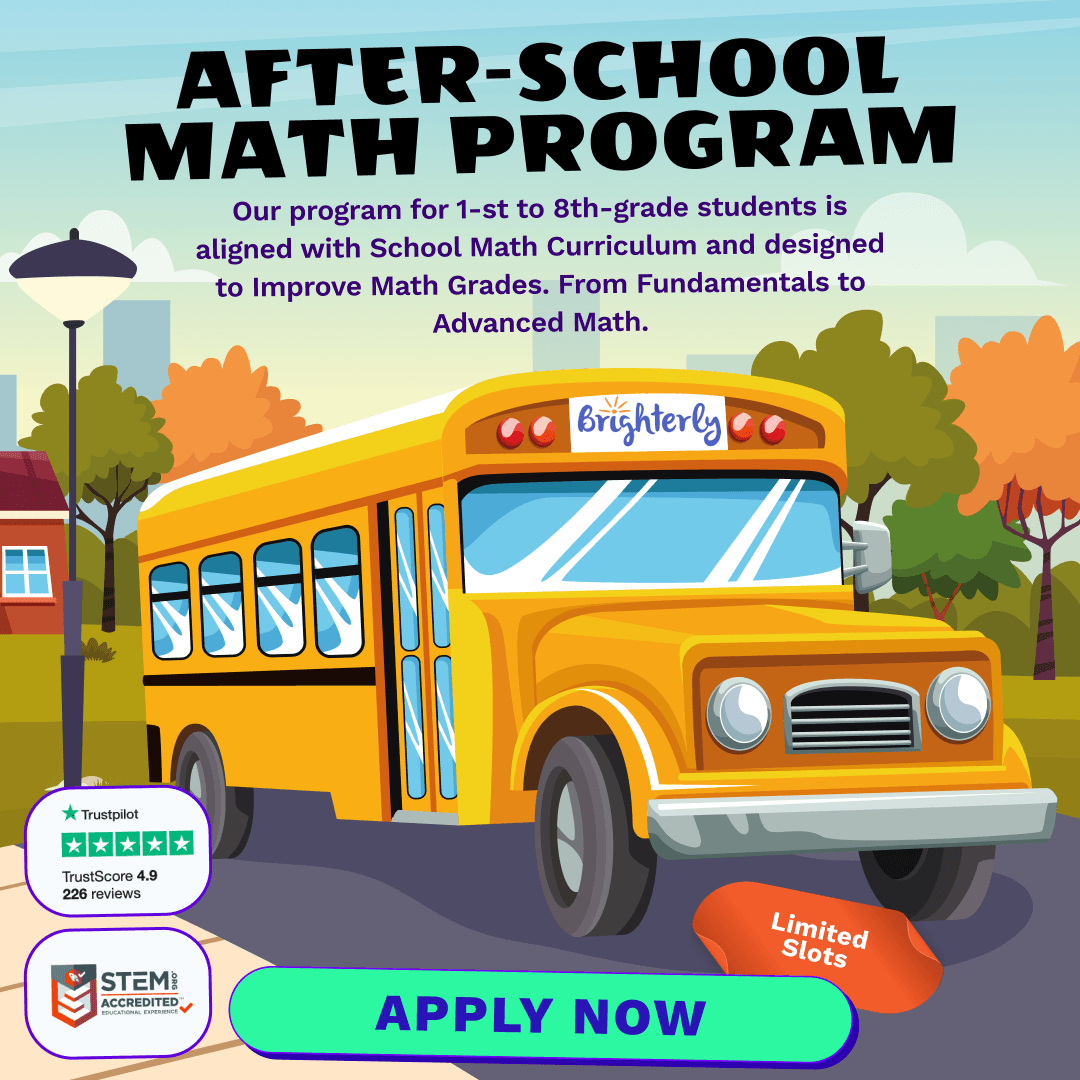# Basic Numbers Math Practice Test for 2nd Grade – [Medium]

Every child’s mathematical journey is filled with exciting milestones, and when you’re in the 2nd grade, the world of numbers becomes even more fascinating. As children progress through their early school years, their understanding of numbers deepens, setting a solid foundation for more advanced math concepts. This article will explore some fundamental 2nd-grade math topics suitable for young learners associated with Brighterly.

## Understanding Place Value

When we look at numbers, it’s essential to understand the position or value of each digit. This concept is known as place value. For instance, in the number 245, the digit 2 is in the hundreds place, 4 is in the tens place, and 5 is in the ones place.

• Units or Ones: The first position from the right.
• Tens: The second position, representing ten times the digit’s value.
• Hundreds: The third position, indicating a hundred times the digit’s value.

Activities such as using base-ten blocks or drawing number rods can be helpful tools to visually understand this concept.

## Expanding and Writing Numbers

One fun exercise for 2nd graders is to expand numbers. This involves breaking down a number based on its place values. For instance:

245 = 200 + 40 + 5

Breaking numbers down this way not only reinforces place value understanding but also paves the way for more advanced arithmetic.

## Odd and Even Numbers

2nd graders are introduced to the difference between odd and even numbers. Simply put:

• Even Numbers: Can be divided by 2 without a remainder. Examples: 2, 4, 6, 8…
• Odd Numbers: Have a remainder when divided by 2. Examples: 1, 3, 5, 7…

Playing games or using interactive math apps can be a great way to recognize and categorize these numbers.

## Basic Arithmetic Operations

The four pillars of arithmetic – addition, subtraction, multiplication, and division – become integral parts of a 2nd grader’s math journey. While multiplication and division are introduced gently, addition and subtraction with bigger numbers (beyond tens) take center stage. Using visual aids, such as number lines or counting beads, can make these processes more intuitive for young learners.

In conclusion, 2nd grade is an adventurous time for budding mathematicians. With resources and activities from platforms like Brighterly, children can have a memorable and educational experience exploring the world of numbers.

Basic Numbers Practice Test for 2nd Grade

Get ready for math lessons with Brighterly! Crafted meticulously to align with essential 2nd-grade math standards, this test offers a balanced blend of challenge and engagement.

1 / 15

What is the place value of 4 in the number 543?

2 / 15

If you add 125 and 75, what is the sum?

3 / 15

Which number is odd?

4 / 15

Which of the following numbers is the smallest?

5 / 15

What is 146 subtracted from 300?

6 / 15

Which number is 10 less than 60?

7 / 15

If you divide 40 by 5, how much do you get?

8 / 15

What is the sum of the digits in the number 256?

9 / 15

What do you get when you multiply 3 by 9?

10 / 15

Which of the following numbers is even and less than 50?

11 / 15

Which number comes next in the sequence: 100, 110, 120, ...?

12 / 15

What is half of 100?

13 / 15

Which number is the closest to 200?

14 / 15

If you have two dimes and three nickels, how much money do you have in cents?

15 / 15

How many tens are there in the number 580?

0%
Poor Level
Weak math proficiency can lead to academic struggles, limited college, and career options, and diminished self-confidence.
Mediocre Level
Weak math proficiency can lead to academic struggles, limited college, and career options, and diminished self-confidence.
Needs Improvement
Start practicing math regularly to avoid your child`s math scores dropping to C or even D.
High Potential
It's important to continue building math proficiency to make sure your child outperforms peers at school.

After-School Math Programs• Our program for 1st to 8th grade students is aligned with School Math Curriculum.

After-School Math Programs
Our program for 1st to 8th grade students is aligned with School Math Curriculum.